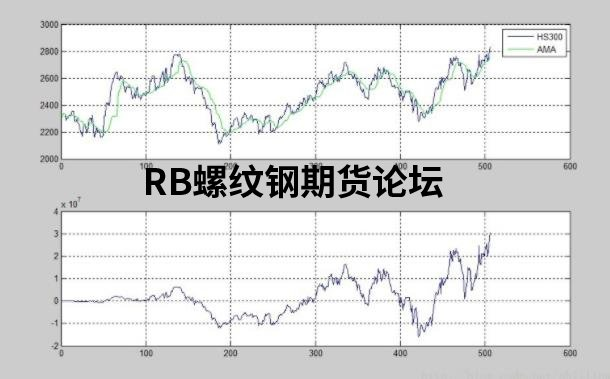# 卡夫曼均线交易系统分享，能更好规避慢趋势和滞后！

AMA的计算公式为：

AMA=AMA+C*(PRICE-AMA)

EMA=EMA+C*(PRICE-EMA)，C=2/(N+1)

AMA的关键在于系数C，要完成抗干扰和滞后性的效果，只需当价格快速单向移动时，将C的值赋值为短周期的指数移动均线的系数，当期货价格成横盘状态时，将C赋值为长周期的指数移动均线的系数即可。

direction = price –price[len];

volatility = @sum(@abs(price –price), n);

Efficiency_Ratio =direction/volativity;

fastest = 2/(N+1) = 2/(2+1) =0.6667;

slowest = 2/(N+1) = 2/(30+1) =0.0645;

smooth = ER*(fastest – slowest)+ slowest;

c = smooth*smooth;

AMA-@lowest(AMA,n)>过滤器，买入

@highest（AMA，n）-AMA<过滤器，卖出1.

`% 卡夫曼自适应移动平均线`

2.

`% Written by Phillip Wan @2013/9/3`

3.

`% Email:hackerwanhappy@foxmail.com`

4.

5.

`% clean work`

6.

`tic;`

7.

`clear;`

8.

`clc;`

9.

`close all;`

10.

`format compact;`

11.

12.

13.

`%% 导入数据`

14.

`Connect = yahoo;`

15.

`Fields = {'Close'};`

16.

`FromDate = '01-Sep-2011';`

17.

`ToDate = '01-Sep-2013';`

18.

`HS300 = fetch(Connect, '000300.SS', Fields, FromDate, ToDate);`

19.

20.

`n=5; %定义区间长度`

21.

`p=0.1; %定义过滤器系数`

22.

`fastlen=30; %定义长期平均周期`

23.

`slowlen=2; %定义短期平均周期`

24.

`w=HS300(:,2);`

25.

`equity=0;`

26.

`equityday=zeros(length(w),1);`

27.

`s=0;`

28.

29.

`ama=zeros(length(w),1);`

30.

`ama(1:n)=w(1:n);`

31.

`for i=n+1:length(w)`

32.

`%% 计算价格方向`

33.

`direction=abs(w(i,1)-w(i-n,1));`

34.

`%% 计算波动性`

35.

`p1=w(i-n:i-1);`

36.

`p2=w(i-n+1:i);`

37.

`vol=sum(abs(p1-p2));`

38.

39.

`if vol~=0`

40.

`%% 计算效率系数（ER）`

41.

`er=direction/vol;`

42.

`fast=2/(fastlen+1);`

43.

`slow=2/(slowlen+1);`

44.

`smooth=er*(fast-slow)+slow;`

45.

`c=smooth*smooth;`

46.

`%% 计算AMA`

47.

`ama(i)=ama(i-1)+c*(w(i,1)-ama(i-1));`

48.

`else`

49.

`ama(i)=ama(i-1);`

50.

`end`

51.

52.

`%% 设置过滤器`

53.

`amaminus=zeros(n-1,1);`

54.

`for t=i-n+1:i`

55.

`amaminus(t,1)=ama(t,1)-ama(t-1,1);`

56.

`end`

57.

`k=p*std(amaminus);`

58.

59.

`%% 根据过滤器进行交易`

60.

`if ama(i)-min(ama(i-n:i))>k`

61.

`s=s+1;`

62.

`equity=equity-w(i)*300;`

63.

`else if max(ama(i-n:i))-ama(i)<k`

64.

`s=s-1`

65.

`equity=equity+w(i)*300;`

66.

`end`

67.

`end`

68.

`equityday(i,1)=equity+s*w(i)*300;`

69.

`end`

70.

71.

72.

`%% 作图`

73.

`figure;`

74.

`subplot(2,1,1);`

75.

`plot(HS300(:,2));`

76.

`hold on;`

77.

`grid on;`

78.

`plot(ama,'g');`

79.

`legend('HS300','AMA');`

80.

`subplot(2,1,2);`

81.

`plot(equityday);`

82.

`grid on;`

## RB螺纹钢期货交易网 专业 全面

### 注册• 回顶部
• 评论